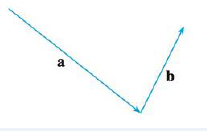Chapter 12, Problem 2RE

Chapter
Section
Textbook Problem

# Copy the vectors in the figure and use them to draw each of the following vectors.(a) a + b (b) a − b (c) − 1 2 a (d) 2a + b(a)

To determine

To sketch: The vector a+b.

Explanation

Definition used:

Triangle law:

Consider the two vectors u and v. Draw vector u and then the second vector, v, at the end of vector u. Then, the resultant vector that connects both the vectors is the sum of the two vectors (u+v).

Graph:

Draw vector a and vector b with the initial point of b as the end point of vector a.

Connect a line between the initial point of vector a and the terminal point of vector b in a to b direction

(b)

To determine

To sketch: The vector ab.

(c)

To determine

To sketch: The vector 12a.

(d)

To determine

To sketch: The vector 2a+b.

### Still sussing out bartleby?

Check out a sample textbook solution.

See a sample solution

#### The Solution to Your Study Problems

Bartleby provides explanations to thousands of textbook problems written by our experts, many with advanced degrees!

Get Started